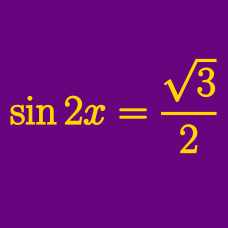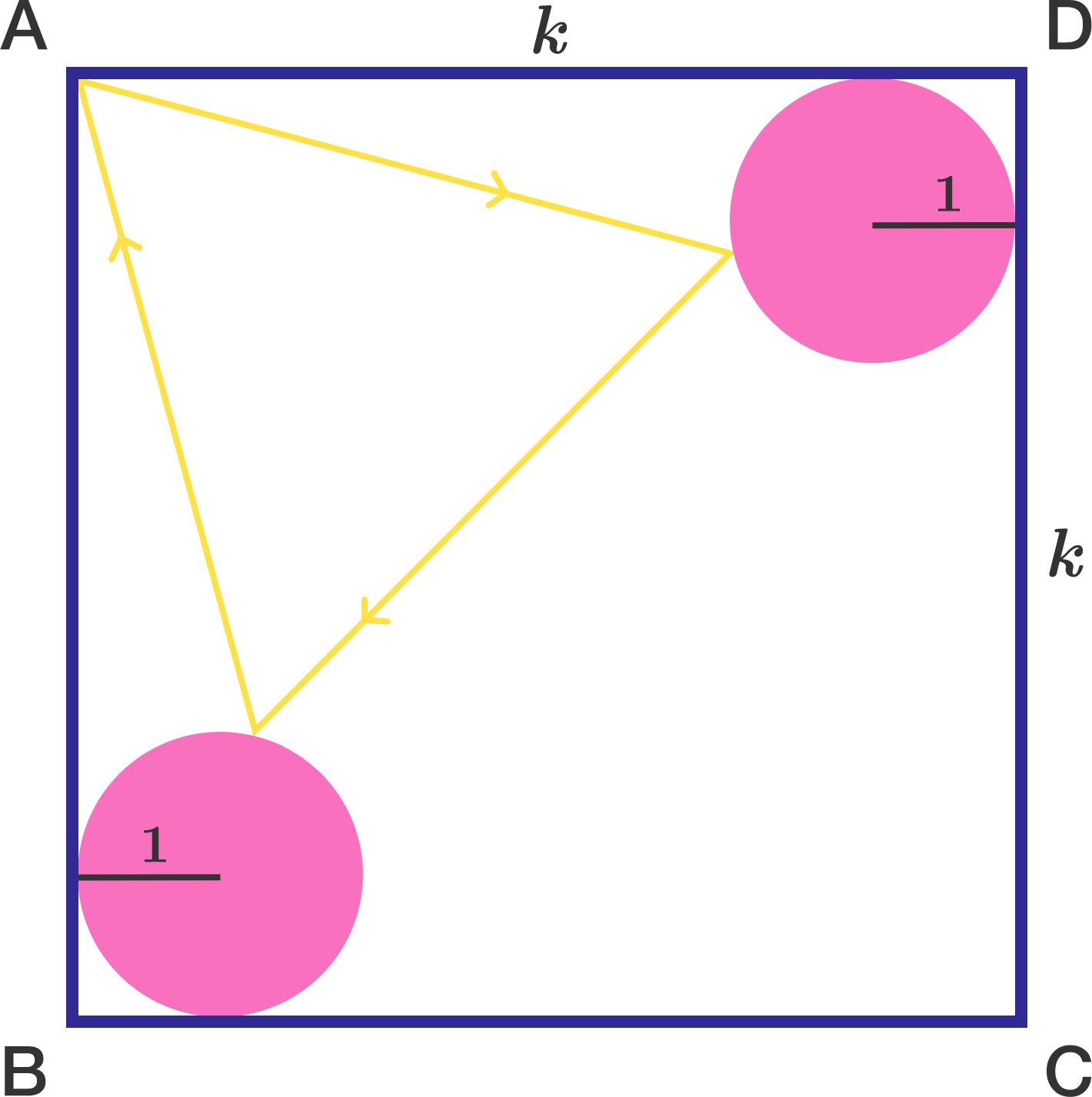Geometry

# Trigonometric Equations: Level 4 Challenges

Find the smallest possible positive value of $\theta$ such that $\tan^2(\theta)=\sec (\theta).$

If $\theta$ can be represented by $\tan^{-1}\left( \ \sqrt{\dfrac{a+\sqrt{b}}{c}}\ \right)$ where $b$ is square free and $a,b,c$ are integers. Find $a+b+c$.

How many real numbers $x$ satisfy

$\pi \cdot \sin x= 2x?$

A square $ABCD$ of side length $k$ contains unit circles at each of corners $B$ and $D$ such that each circle is tangent to the square at precisely two points. A ray of light emanating from point $A$ reflects off each circle and then returns to $A$, creating a path in the shape of an equilateral triangle.

There is a unique value of $k$ for which this scenario can occur. Find $\lfloor 10000\cdot k \rfloor$.

Note: "Reflecting" means that the angle of incidence equals the angle of reflection.It is the case that $S = \displaystyle\sum_{n=-1}^{2015} \csc((2^{n+4})^{\circ}) = \sec(k^{\circ})$ for some integer $0 \lt k \lt 90$. Find $k$.

Find the sum of all $\theta$ such that $\sin ^8 {\theta} + \cos ^8 {\theta} = \frac{17}{32} \quad \text{where } 0 \leq \theta \leq \pi.$

×